# Steady-State Solution of MHD Heat and Mass Transfer Fluid Flow over a Semi-Infinite Vertical Plate in a Rotating System Dipped in a Porous Medium with Hall Current, Thermal Radiation, Heat Generation/Absorption and Joule Heating

Steady-State Solution of MHD Heat and Mass Transfer Fluid Flow over a Semi-Infinite Vertical Plate in a Rotating System Dipped in a Porous Medium with Hall Current, Thermal Radiation, Heat Generation/Absorption and Joule Heating

Saykat Poddar* Muhammad Minarul Islam Jannatul Ferdouse Md. Mahmud Alam

Department of Mathematics, Faculty of Science, Bangabandhu Sheikh Mujibur Rahman Science and Technology University, Gopalganj 8100, Bangladesh

Mathematics Discipline, Science, Engineering and Technology School, Khulna University, Khulna 9208, Bangladesh

Corresponding Author Email:
saykat.sp@gmail.com
Page:
457-467
|
DOI:
https://doi.org/10.18280/ijht.400213
25 June 2020
|
Accepted:
11 March 2021
|
Published:
30 April 2022
| Citation

OPEN ACCESS

Abstract:

The steady-state solution of unsteady MHD radiating fluid flow concerning heat and mass transfer in a rotating system dipped in a medium of porosity with Hall current along a semi-infinite vertical plate have been inquired numerically. By using appropriate usual conversions, the governing equations for the present study are converted into the form of dimensionless non-linear partial differential equations (PDEs). The explicit finite difference method (FDM) is imposed to solve the acquired coupled non-linear PDEs. MATLAB R2020a has been employed as a numerical simulation tool. For assuring accuracy and for obtaining convergence solutions the stability and convergence criteria of the present model have been fabricated. The stability analysis imposes circumscriptions on several elements as 0.50≤M≤10, 0.01≤βh≤5, K≤326.50, 0.185≤Pr≤7.56, 0.00≤R*≤80, Sc≥0.18 and Q0≤326.50. The mesh sensitivity experiment for finer mesh space and time validation experiment for steady-state solutions are comprised of acquired solutions. The consequences of the present findings are compared with several previously published research works for claiming validation. The present study accentuates the effects of those parameters, which are relevant to the dimensionless model equations. Finally, the obtained results are placed in graphs and sorted into tables.

Keywords:

MHD, porous medium, finite difference method (FDM), hall current

1. Introduction

Magneto-hydrodynamics (MHD) principles have been equipped for umpteen engineering purposes such as dynamo, fusion reactors, motor mechanisms, and flow meters, etc. Biomedical sciences also have huge applications of MHD. The heat transfer and mass transfer occurrence through homogeneous medium of porosity have gained noticeable intentness due to their manifold significant applications in geophysics and energy-connected problems. Heat transfer in boundary layer flow has extensive uses in production-related engineering. In recent decades, the flow behavior via a porous medium is getting vast attention in the applied area of subterranean water resources, petroleum technology, and agricultural engineering to look for the motion of natural gas, oil, and filtration system in the field of ocean engineering. In geophysical and geothermal fields the characteristics of fluid flows via the porous medium in a rotating environment have meaningful influences. It has also notable importance in solar physics dealt with the solar cycle and the interior construction of circularly moving stars.

Sattar and Alam  investigated the hydro-magnetic flow nature of incompressible fluid with Hall current by considering a vertically oriented porous plate with the feature of time dependency. The cross-flow was observed and negligible responses were found on the primary velocity for the Hall impact whereas the secondary velocity shows hiking for it. Biswal and Sahoo  presented brilliant research on the mass transfer phenomena of oscillatory MHD viscoelastic flow with Hall current. The influence of temperature on viscosity of mass transfer flow on a hydrogen-air mixture with Dufour and Soret effects has been investigated by Kafoussias and Williams . Repercussions of Hall current and ion-slip formation on hydromagnetic flow by engaging heat transfer occurrence was researched by Takhar and Jha . Dissipative effect on MHD flow past a cylindrical duct with buoyancy effect was checked by Barletta and Rossi di Schio . Anwar Be´g et al.  reported on the numerical reckoning of MHD non-Newtonian flow with the involvement of a wedge embedded in the porous environment of Darcy-Brinkman type. A study incorporating the responses of Joule heating on MHD flow of gaseous ion with isothermally radiating surfaces has been investigated by Duwairi . The inclusion of dissipative impact was mentioned as the reason behind the reduction of heat transfer rate. Alam et al.  iteratively counted mixed convection boundary layer flow by considering the generation of heat along with the thermal diffusion effect. Complete scrutiny on stability analysis for MHD flow of Casson and Maxwell fluid has been represented by Reza-E-Rabbi et al. . Sharma et al.  perused the Hall current by noticing heat source or sink impacts on transient MHD flow. Alam et al.  checked steady MHD mass transfer flow with thermophoresis by taking variable suction into account. Intensive application of group theoretical approach on MHD flow wielded in a saturated permeability medium with radiative impacts was accomplished by Bakier et al. . They reported that the radiative phenomena lead the stream function to be hiked up. Chemical reaction effect along with buoyancy on mixed convective MHD flow with the existence of thermal radiation and Ohmic heating have been reviewed by Pal and Talukder .

Alam et al.  checked joule heating and dissipative impacts on MHD steady flow driven into a permeability medium with rotation. Islam and Islam  inquired hydromagnetic micropolar fluid flow past a stretching wedge by imposing the Nachtsheim-Swigert shooting scheme. MHD flow nature past an isothermal plate of vertical position with the appearance of heat transfer and heat generation/absorption has been researched by Reddy et al. . Interlocution of the mechanics of bifurcation and heat transfer was executed by Hasan et al. . Seth et al.  observed exact solution of transient MHD radiating flow immersed in a porous environment with rotation. Rashad et al.  precisely inquired on the laminar nanofluid flow through a vertically oriented cylinder by adopting Brownian motion as well as thermophoresis into consideration. Laplace and inverse Laplace transformations technique on transient MHD radiating and chemically reactive fluid flow by involving the oscillatory motion of the free-stream have been reckoned by Seth et al. . Raju et al.  executed thorough scrutiny on radiation effect of MHD flow behavior in a permeability medium subjected by an upright surface. Singh et al.  established transient MHD flow features through a plate of oscillation in the vertical position by considering Hall and ion-slip current. Changes in viscosity due to magnetic influence has been quested by Izadi et al.  for micropolar nanofluidic flow under MHD phenomena. Veera Krishna et al.  established a broad perscrutation on the thermal diffusion and Joule heating impacts of viscous MHD flow with the consideration of Hall current and rotation. They reported an increment in flow velocity for the Hall phenomena and buoyancy impacts. Islam et al. [25, 26] and Mollah et al.  inquired about unsteady viscous compressible or incompressible flow behavior with the generation of magnetic field induction by using numerical schemes. Also, Mollah et al.  have considered Hall current along with ion-slip effects on transient MHD non-Newtonian flow of Bingham rheology between parallel plates where explicit FDM was used as solving mechanism. Further, a study related to the EMHD Bingham fluid flow within parallel Riga plates has been inspected by Mollah . Hall Impact on MHD flow for miscellaneous geometries with or without system rotation is studied in refs. [30-32].

All the above investigations covering different case studies on the Navier-Stokes’ equations, heat transfer, and/or mass transfer in several different circumstances. Considering those contexts, impacts of Hall current, thermal radiation, heat generation/absorption along Joule heating forces are employed to inspect the flow pattern of the current study under the effect of the influencing parameters in a rotating porous medium. This study also deals with the unsteady heat transfer and species mass transfer phenomena. The FDM [33, 34] algorithm have been conducted for the numerical inquiry of the dimensionless non-linear PDEs.

2. Mathematical Model Establishment

Assume an unsteady heat and mass transfer flow of MHD viscous electrically conducting incompressible fluid over an electrically non-conducting semi-infinite vertical plate with a stationary position in a porous medium where the system is deemed to be rotating with negative uniform angular velocity Ωr about an axis normal to the plate. The rotation of angular velocity is supposed to be in the clockwise direction. Only Coriolis acceleration is engaged in this study and centripetal acceleration has a futile impact since it is wee in magnitude. The x*-axis is planned to set in the direction of the plate, which is chosen in the favorable flow direction and the y*-axis is occupied perpendicular to the plate. A strong magnetic field B=(0, B0, 0) is imposed perpendicular to the plate. The induced magnetic field is out of consideration here, since inadequate values of magnetic Reynolds number have arisen for the flowing fluids. Initially, in the sense of time, it is deemed that the plate along with the fluid is at the identical temperature $T_{\infty}^{*}$ and concentration level $C_{\infty}^{*}$. Then, at the time $t^{*}>0$, the temperature and concentration level at the plate is assumed as $T_{w}^{*}$ and $C_{w}^{*}$, where $T_{\infty}^{*}$ and $C_{\infty}^{*}$ are the quiescent region temperature and species concentration. Now let, q=(u*, v*, w*) as the fluid velocity vector and $J=\left(J_{x^{*}}, J_{y^{*}}, J_{z^{*}}\right)$ as the current density vector. The representation of conservation of charge ∇.J=0 generates $J_{y^{*}}$=constant. The modified form of the generalized Ohm’s law by holding Hall term is , J=σ[E+q×B+β(J×B)], where, β is the Hall factor. On simplification, it generates:

${{J}_{{{x}^{*}}}}=-\frac{\sigma {{B}_{0}}}{\left( 1+\beta _{h}^{2} \right)}({{w}^{*}}-{{\beta }_{h}}{{u}^{*}})$ and ${{J}_{{{z}^{*}}}}=\frac{\sigma {{B}_{0}}}{\left( 1+\beta _{h}^{2} \right)}({{u}^{*}}+{{\beta }_{h}}{{w}^{*}})$

where, σ is the electrical conductivity and βh=σβB0 is the Hall parameter. The impacts of ion-slip along with thermo-electric effects are not comprised and the electron pressure gradient is neglected. Also, the electric field intensity is contemplated to be ineffective since the plate is non- conducting. The schematic flow region and coordinate system of the model are depicted in Figure 1.

## 1.png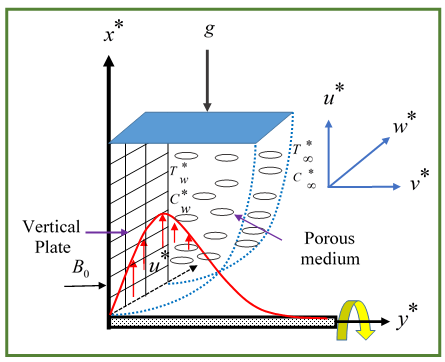Figure 1. Schematic model and coordinate system

By the above-narrated assumptions, the governing system of coupled non-linear PDEs are mentioned below [14, 34]:

Continuity equation:

$\frac{\partial {{u}^{*}}}{\partial {{x}^{*}}}+\frac{\partial {{v}^{*}}}{\partial {{y}^{*}}}=0$                (1)

The momentum equations:

\begin{align} & \frac{\partial {{u}^{*}}}{\partial {{t}^{*}}\,}+{{u}^{*}}\frac{\partial {{u}^{*}}}{\partial {{x}^{*}}}+{{v}^{*}}\frac{\partial {{u}^{*}}}{\partial {{y}^{*}}}=\upsilon \frac{{{\partial }^{2}}{{u}^{*}}}{\partial {{y}^{*}}^{2}}-\frac{\sigma B_{0}^{2}}{\rho \left( 1+\beta _{h}^{2} \right)}({{u}^{*}}+{{\beta }_{h}}{{w}^{*}}) \text{ }+g\beta ({{T}^{*}}-T_{\infty }^{*})+g{{\beta }^{*}}({{C}^{*}}-C_{\infty }^{*})-\frac{\upsilon }{{{k}_{p}}}u-2{{\Omega }_{r}}{{w}^{*}} \\ \end{align}           (2)

\begin{align} & \frac{\partial {{w}^{*}}}{\partial {{t}^{*}}\,}+{{u}^{*}}\frac{\partial {{w}^{*}}}{\partial {{x}^{*}}}+{{v}^{*}}\frac{\partial {{w}^{*}}}{\partial {{y}^{*}}}=\upsilon \frac{{{\partial }^{2}}{{w}^{*}}}{\partial {{y}^{*}}^{2}} \text{ }-\frac{\sigma B_{0}^{2}}{\rho \left( 1+\beta _{h}^{2} \right)}({{w}^{*}}-{{\beta }_{h}}{{u}^{*}})-\frac{\upsilon }{{{k}_{p}}}{{w}^{*}}+2{{\Omega }_{r}}{{u}^{*}} \\\end{align}               (3)

The energy equation:

\begin{align} & \frac{\partial {{T}^{*}}}{\partial {{t}^{*}}\,}+{{u}^{*}}\frac{\partial {{T}^{*}}}{\partial {{x}^{*}}}+{{v}^{*}}\frac{\partial {{T}^{*}}}{\partial {{y}^{*}}}=\frac{\kappa }{\rho {{c}_{p}}}\frac{{{\partial }^{2}}{{T}^{*}}}{\partial {{y}^{*}}^{2}} \text{ }+\frac{\upsilon }{{{c}_{p}}}\left[ {{\left( \frac{\partial {{u}^{*}}}{\partial {{y}^{*}}} \right)}^{2}}+{{\left( \frac{\partial {{w}^{*}}}{\partial {{y}^{*}}} \right)}^{2}} \right]-\frac{{{Q}^{*}}}{\rho {{c}_{p}}}\left( {{T}^{*}}-T_{\infty }^{*} \right) \text{ }+\frac{\sigma B_{0}^{2}}{\rho {{c}_{p}}(1+\beta _{h}^{2})}\left( {{u}^{*}}^{2}+{{w}^{*}}^{2} \right)+\frac{1}{\rho {{c}_{p}}}\frac{16{{\sigma }^{*}}}{3{{k}^{*}}}T{{_{\infty }^{*}}^{3}}\frac{{{\partial }^{2}}{{T}^{*}}}{\partial {{y}^{*}}^{2}} \\ \end{align}                (4)

The concentration equation:

$\frac{\partial {{C}^{*}}}{\partial {{t}^{*}}\,}+{{u}^{*}}\frac{\partial {{C}^{*}}}{\partial {{x}^{*}}}+{{v}^{*}}\frac{\partial {{C}^{*}}}{\partial {{y}^{*}}}={{D}^{*}}\frac{{{\partial }^{2}}{{C}^{*}}}{\partial {{y}^{*}}^{2}}+\frac{{{D}^{*}}{{k}_{{{T}^{*}}}}}{T_{m}^{*}}\frac{{{\partial }^{2}}{{T}^{*}}}{\partial {{y}^{*}}^{2}}$                     (5)

The respective initial and boundary conditions are:

\left. \begin{align} & {{t}^{*}}\le 0; \\ & {{u}^{*}}=0,{{v}^{*}}=0,{{w}^{*}}=0,{{T}^{*}}\to T_{\infty }^{*},{{C}^{*}}\to C_{\infty }^{*}\text{ everywhere} \\ \end{align} \right\}               (6)

\left. \begin{align} & {{t}^{*}}>0; {{u}^{*}}=0,{{v}^{*}}=0,{{w}^{*}}=0,{{T}^{*}}\to T_{\infty }^{*},{{C}^{*}}\to C_{\infty }^{*}\text{ at }{{x}^{*}}=0 {{u}^{*}}=0,{{v}^{*}}=0,{{w}^{*}}=0,{{T}^{*}}=T_{w}^{*},{{C}^{*}}=C_{w}^{*}\text{ at }{{\text{y}}^{*}}=0 \\ & {{u}^{*}}=0,{{v}^{*}}=0,{{w}^{*}}=0,{{T}^{*}}\to T_{\infty }^{*},{{C}^{*}}\to C_{\infty }^{*}\text{ at }{{y}^{*}}\to \infty \\ \end{align} \right\}              (7)

Since the explicit FDM is applied to solve the non-linear dimensionless PDEs (1)-(5) so, it is mandatory to construct these equations dimensionless.

For doing this, the following normalized quantities have been employed where L(m) is length scale, q*(m/s) is fluid velocity scale, T*(K) is temperature scale and C*(kg/m3) is concentration scale:

\left. \begin{align} & t=\frac{\upsilon {{t}^{*}}}{{{L}^{2}}},x=\frac{{{x}^{*}}}{L},y=\frac{{{y}^{*}}}{L},u=\frac{{{u}^{*}}L}{\upsilon },v=\frac{{{v}^{*}}L}{\upsilon }, \\ & w=\frac{{{w}^{*}}L}{\upsilon },T=\frac{{{T}^{*}}-T_{\infty }^{*}}{T_{w}^{*}-T_{\infty }^{*}},C=\frac{{{C}^{*}}-C_{\infty }^{*}}{C_{w}^{*}-C_{\infty }^{*}} \\ \end{align} \right\}              (8)

The retrieved dimensionless non-linear PDEs are:

$\frac{\partial u}{\partial x}+\frac{\partial v}{\partial y}=0$               (9)

\begin{align} & \frac{\partial u}{\partial t}+u\frac{\partial u}{\partial x}+v\frac{\partial u}{\partial y} =\frac{{{\partial }^{2}}u}{\partial {{y}^{2}}}+T+C-Ku-{{R}_{o}}w-\frac{M}{\left( 1+\beta _{h}^{2} \right)}(u+{{\beta }_{h}}w) \\ \end{align}                       (10)

$\frac{\partial w}{\partial t}+u\frac{\partial w}{\partial x}+v\frac{\partial w}{\partial y}=\frac{{{\partial }^{2}}w}{\partial {{y}^{2}}}-Kw+{{R}_{o}}u-\frac{M}{\left( 1+\beta _{h}^{2} \right)}(w-{{\beta }_{h}}u)$                (11)

\begin{align} & \frac{\partial T}{\partial t\,}+u\frac{\partial T}{\partial x}+v\frac{\partial T}{\partial y}=\left( \frac{2+{{R}^{*}}}{2{{P}_{r}}} \right)\frac{{{\partial }^{2}}T}{\partial {{y}^{2}}} +{{E}_{c}}\left[ {{\left( \frac{\partial u}{\partial y} \right)}^{2}}+{{\left( \frac{\partial w}{\partial y} \right)}^{2}} \right]-{{Q}_{0}}T+\frac{{{J}_{h}}}{(1+\beta _{h}^{2})}\left( {{u}^{2}}+{{w}^{2}} \right) \\ \end{align}              (12)

$\frac{\partial C}{\partial t\,}+u\frac{\partial C}{\partial x}+v\frac{\partial C}{\partial y}=\frac{1}{{{S}_{c}}}\frac{{{\partial }^{2}}C}{\partial {{y}^{2}}}+{{S}_{r}}\frac{{{\partial }^{2}}T}{\partial {{y}^{2}}}$              (13)

The non-dimensional approach has disclosed that the characteristic length L can be taken as:

$L={{\left[ \frac{{{\upsilon }^{2}}}{g\beta \left( T_{w}^{*}-T_{\infty }^{*} \right)} \right]}^{\frac{1}{3}}}={{\left[ \frac{{{\upsilon }^{2}}}{g{{\beta }^{*}}\left( C_{w}^{*}-C_{\infty }^{*} \right)} \right]}^{\frac{1}{3}}}$

The dimensionless conditions take the following form:

\left. \begin{align} & t\le 0; \\ & u=0,v=0,w=0,T\to 0,C\to 0\text{ everywhere} \\ \end{align} \right\}                  (14)

\left. \begin{align} & t>0; \\ & u=0,v=0,w=0,T\to 0,C\to 0\text{ at }x=0 \\ & u=0,v=0,w=0,T=1,C=1\text{ at y}=0 \\ & u=0,v=0,w=0,T\to 0,C\to 0\text{ at }y\to \infty \\ \end{align} \right\}                (15)

The above described non-dimensional parameters and numbers can be noted as the following forms below:

$M=\frac{\sigma B_{0}^{2} L^{2}}{\rho v}$ (magnetic parameter), $P_{r}=\frac{v c_{p} \rho}{\kappa} \quad$ (Prandtl number), $\quad R^{*}=\frac{32 \sigma^{*} T_{\infty}^{* 3}}{3 \kappa k^{*}}$  (radiation parameter), $R_{o}=\frac{2 \Omega_{r} L^{2}}{v}$ (rotational parameter), $E_{c}=\frac{v^{2}}{c_{p}\left(T_{w}^{*}-T_{\infty}^{*}\right) L^{2}}$ (Eckert number), $Q_{0}=\frac{Q^{*} L^{2}}{\rho c_{p} v}$ (heat generation/absorption parameter), $J_{h}=\frac{\sigma B_{0}^{2} v}{\rho c_{p}\left(T_{w}^{*}-T_{\infty}^{*}\right)}\left(\right.$ Joule heating parameter), $S_{c}=\frac{v}{D^{*}}$ (Schmidt number), $S_{r}=\frac{D^{*} k_{T^{*}}\left(T_{W}^{*}-T_{\infty}^{*}\right)}{v T_{m}^{*}\left(C_{w}^{*}-C_{\infty}^{*}\right)}($ Soret number $), K=\frac{L^{2}}{k_{p}}$ (permeability parameter), and βh=σβB0 is the Hall parameter.

3. Principal Physical Quantities

The quantities which have operative physical meaning in various engineering assignment are narrated in this section. The shear stress coefficient characterizes the nature of the fluid layer contiguous to the boundary (plate). The Nusselt number indicates the measurement of whether the convection process dominates heat transfer or not. In general, large magnitudes of Nusselt number arise when the convection process dominates heat transfer. The Sherwood number indicates the mass transfer coefficient in a mass transport system. The shear stress towards primary velocity direction adjacent to the boundary (plate) and the shear stress towards secondary velocity direction adjacent to the boundary (plate) are respectively known as primary and secondary shear stress and can be defined as $\tau_{x L}=\mu\left(\frac{\partial u^{*}}{\partial y^{*}}\right)_{y^{*}=0}$ and $\tau_{z L}=\mu\left(\frac{\partial w^{*}}{\partial y^{*}}\right)_{y^{*}=0}$ which in terms of non-dimensional criteria take the form $\left(\frac{\partial u}{\partial y}\right)_{y=0}$ and $\left(\frac{\partial w}{\partial y}\right)_{y=0}$ respectively. In terms of non-dimensional criteria, the local Nusselt number and local Sherwood number can be counted from temperature and concentration distributions respectively as $N u_{L}=-\left(\frac{\partial T}{\partial y}\right)_{y=0}$ and $S h_{L}=-\left(\frac{\partial C}{\partial y}\right)_{y=0}$.

4. Numerical Discretization Technique

The normalized system of Eqns. (9)-(13) is discretized in time with the Euler time integral where the earlier time step values are imported to reckon the new time step values, in an explicit manner . The space discretization is performed with the backward differencing in the first-order derivative and central differencing is considered for higher- order [33, 34]. The FDM algorithm is implemented in MATLAB with the boundary conditions (14) and (15). The vertical plate of the model is considered of height x(=100)max and regarding y(=35)max. These ranges of the coordinates are chosen arbitrarily, just keeping in mind that the height is bigger, compared to the width for the setup model. The mesh spacing is envisaged as m=150 and n=150 in the x and y directions respectively as demonstrated in Figure 2. This uniform spacing is chosen in a trial and error manner. We are deeming that Δx and Δy are constant cell sizes in x and y directions respectively and can be chosen as:

$\Delta x=0.6667\left( 0\le x\le 100 \right)$

$\Delta y=0.2333\left( 0\le y\le 35 \right)$

along with a tiny Euler time-stepping, Δt=0.005.

## 2.png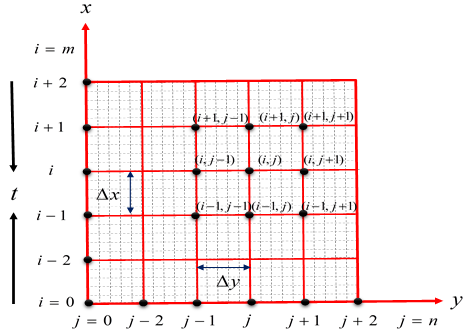Figure 2. Finite difference space grids

5. Stability

Explicit FDM requires stability and convergence criteria  to ensure the validity and converging nature of the solutions. With the established stability criteria, the steady-state profiles are obtained and a finer mesh setup is found. In present status, the stability conditions on discretized equations can be specified as:

$u\frac{\Delta t}{\Delta x}-\left| v \right|\frac{\Delta t}{\Delta y}+\frac{2\Delta t}{{{\left( \Delta y \right)}^{2}}}+K\frac{\Delta t}{2}+\frac{M}{\left( 1+\beta _{h}^{2} \right)}\frac{\Delta t}{2}\le 1$                  (16)

$u\frac{\Delta t}{\Delta x}-\left| v \right|\frac{\Delta t}{\Delta y}+\left( \frac{2+{{R}^{*}}}{2{{P}_{r}}} \right)\frac{2\Delta t}{{{\left( \Delta y \right)}^{2}}}+{{Q}_{0}}\frac{\Delta t}{2}\le 1$                (17)

$u\frac{\Delta t}{\Delta x}-\left| v \right|\frac{\Delta t}{\Delta y}+\frac{1}{{{S}_{c}}}\frac{2\Delta t}{{{\left( \Delta y \right)}^{2}}}\le 1$               (18)

The values Δt=0.005, Δy=0.2333, and initial conditions generate expected restrictions as 0.50≤M≤10, 0.01≤βh≤5, K≤326.50, 0.185≤Pr≤7.56, 0.00≤R*≤80, Sc≥0.18 and Q0≤326.50 with Ec=0.01.

6. Findings and Discussions

The heat and mass transfer phenomena on MHD flow are studied with the consideration of Hall current, Joule heating, heat generation/absorption, and thermal radiation. The assumption of a porous medium with a rotating environment is also addressed in this work. Simplifications of the governing equations are performed under boundary layer approximations. The reckoning of numerical performances for dimensionless primary velocity u, secondary velocity w, temperature distribution T and concentration C have been calculated within the flow region to make a judgment about the developed physical model. Appropriate mesh space (see section 6.1) is found and the steady-state of the solutions (see section 6.2) has been analyzed. The impacts of several affined parameters such as magnetic parameter (M), heat changing parameter (Q0), radiation parameter (R*), Prandtl number (Pr), rotational parameter (Ro), Joule heating parameter (Jh), Schmidt number (Sc), Eckert number (Ec), Soret number (Sr), Hall parameter (βh), and permeability parameter (K) on primary velocity, secondary velocity, temperature profile, concentration profile along with local primary shear stress, local secondary shear stress, local Nusselt number, and local Sherwood number have been portrayed and discussed.

A tabular type validation checking of this work with published numerical and analytical investigations has been represented with the impact of the magnetic parameter (M).

6.1 Mesh sensitivity experiment

Mesh sensitivity experiment stands for the findings of precise mesh size i.e., the congruous value of m and n. For searching competent mesh size, the experimentation is carried out for some distinct values, m=120, n=120; m=140, n=140 and m=160, n=160 as portrayed in Figure 3, where, M=1.00, Pr=0.71, βh=0.50, K=1.00, Ro=0.60, R*=0.30, Ec=0.01, Jh=1.00, Q0=0.80, Sc=0.30 and So=0.20 at the time t=120 (steady-state). The obtained curves are converging in nature and show an ineffective variation among them. With reference to the above-counted values of meshes, for further calculations and estimations, the mesh sizes m=150, n=150 are picked.

## 3.png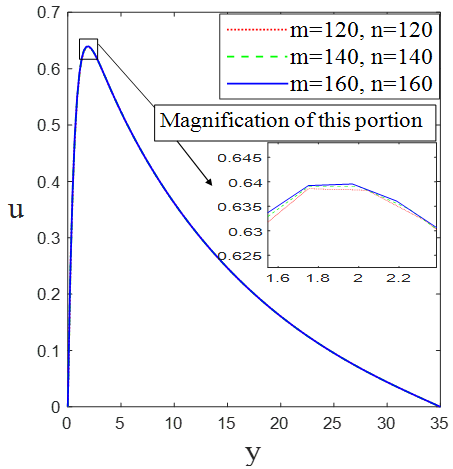Figure 3. Portray of mesh sensitivity for primary velocity

6.2 Time sensitivity experiment

Time sensitivity experiment stands for the findings of steady-state for primary velocity, secondary velocity, temperature distribution, and species concentration profile. To do so, the experimentation has been computed for several dimensionless Euler time-stepping quantities such as t=10, 20, 30, 40, 50, 60, 70, 80, 90, 100, 110, 120 and 130. It is marked from Figure 4, that, the attained results expose minor variation after t=90 for all profiles and exhibit ineffective variation forthwith after t=120. Hence, for numerical computations, the steady-state solutions are confirmed at the time t=120. Precise observation discloses that Temperature distribution attains steady-state in a faster manner compared to other profiles. The nature of variations in primary velocity, secondary velocity, and concentration distribution among these time steps are quite similar.

6.3 Validation checking with published results

Substantial scrutiny on the impacts of Hall current and Joule heating on MHD boundary layer flow has been examined with thermal radiation in the current paper. Analyzing the comparison of this paper with published papers is important for claiming validation of the retrieved results. Comparison of the consequences involving the current study with several published numerical and analytical studies have been embodied in Table 1. For the purpose of conducting validation checking, the works of Alam et al. , Veera Krishna et al. , and Seth et al.  have been selected. All these works which are mentioned here, are researched under the existence of a magnetic field. For the sake of validation checking with brevity, we have shown the impact of the magnetic parameter only. The study of Alam et al.  is based on the Nachtsheim-Swigert iterative shooting method, whereas perturbation technique and Laplace transformation have been employed as solution procedure in the studies of Veera Krishna et al.  and Seth et al.  respectively.

## 4a.png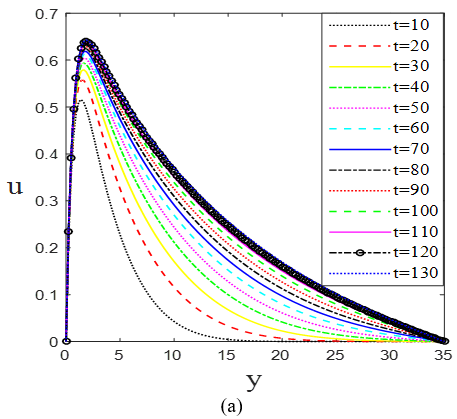## 4b.png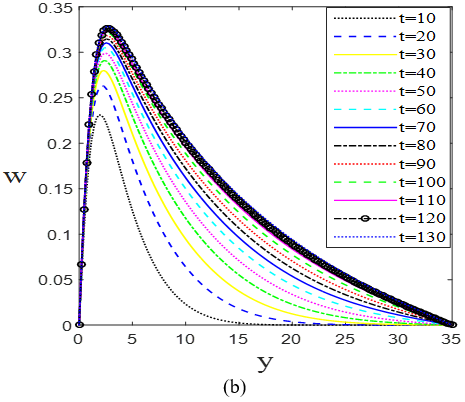## 4c.png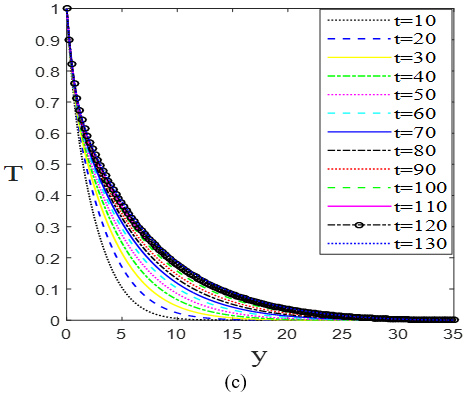## 4d.png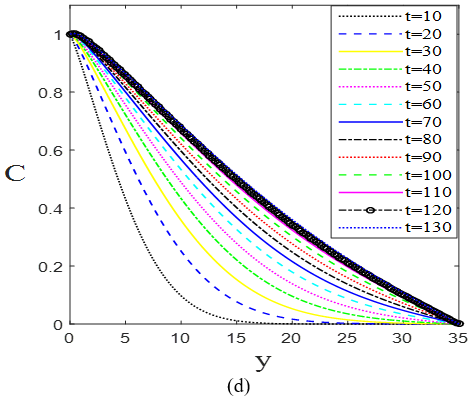Figure 4. Portrays of time sensitivity for (a) primary velocity (b) secondary velocity; (c) temperature profile and (d) concentration profile

Table 1. Comparison analyze

 Output Effect on Present result Alam et al.  Veera Krishna et al.  Seth et al.  Magnetic parameter M u (Dec.) (Dec.) (Dec.) (Dec.) w (Dec.) (Inc.) (Inc.) (Dec.) T (Dec.) -- (Dec.) -- C (Neglig-ible) -- -- -- τxL (Dec.) (Dec.) (Dec.) -- τzL (Dec.) (Inc.) -- -- NuL (Inc.) -- (Inc.) -- ShL (Dec.) -- (Dec.) -- Dec. means Decreasing; Inc. means Increasing

6.4 Influences of relevant parameters

In general, adopting the values of M=1.00, Pr=0.71, βh=0.50, K=1.00, Ro=0.60, R*=0.30, Ec=0.01, Jh=1.00, Q0=0.80, Sc=0.30, So=0.20 at the time t=120 (steady-state), the impacts of sundry values of relevant parameters on primary velocity u, secondary velocity w, temperature distribution T and concentration profile C have been discussed in Figures 5-9. For brevity, two individual parameters impact are portrayed in a single graph. The motivation for the choice of sundry values for the Hall parameter βh is based on refs. [30-34]. The concentration profile will be portrayed for the Soret number So and Schmidt number Sc only, because the rest of the parameters reveal negligible variations on it. For a concise view, the impacts of parameters on local primary shear stress τxL, local secondary shear stress τzL, local Nusselt number NuL, and local Sherwood number ShL have been embodied in Table 2. This study is kept limited for Pr=0.71 (exhibits air at 20℃), Pr=1.00 (exhibits electrolytic solution at 20℃), and Pr=7.00 (exhibits water at 20℃) because these are the most significant common fluids. The rest of the parameters are selected arbitrarily in magnitude by following the stability conditions. Figure 5, delineates that, the primary velocity, secondary velocity, and temperature distribution fall with the augmentation of M as well as all the mentioned profiles reduce with the raise of Pr. Retardation effects on the primary velocity, secondary velocity, and temperature profile with the raise of permeability parameter K are marked in Figure 6. Also, a diminishing influence on the primary velocity and temperature distribution, on contrary an amplifying impact on secondary velocity is reckoned with the increase of Hall parameter βh from same Figure 6. Figure 7, discloses that the primary velocity and temperature profile reduce substantially with the enlargement of the rotational parameter Ro while secondary velocity increases with Ro.

Furthermore, all the primary velocity, secondary velocity, and temperature distribution diminish with the rise of heat generation/absorption parameter Q0 which are represented in the same Figure 7. Figure 8, portrays that, all the primary velocity, secondary velocity, and temperature distribution rise with the augmentation of Joule heating parameter Jh, whereas increasing values of radiation parameter R* expose a gradual increment on them in proximity to the plate, thereafter, become static. It is marked from Figure 9, that, a significant prolongation occurs on all the primary velocity, secondary velocity, temperature distribution and concentration distribution by the promotion of Soret number Sr, simultaneously, Schmidt number Sc occurs demotion in all those profiles.

Table 2 references the influences of several parameters on local primary shear stress, local secondary shear stress, local Nusselt number, and local Sherwood number. Enhancement indication of local primary shear stress with the increment of Jh, βh, Sr, Sc, and R* henceforth, decrement indication with M, Pr, K, Ro, and Q0, is marked. The local secondary shear stress promotes with the promotion of Jh, βh, Sr, Ro, and R* whereas it demotes with M, Pr, K, and Q0. Local Nusselt number raises with M, Pr, K, Ro, βh, Sc, and Q0 on contrary, it collapses with Jh, Sr, and R*. The increasing influence of Jh and R* cause augmentation in local Sherwood number while raise of M, Pr, K, Ro, βh, Sc, Sr, and Q0 cause retardation in it.

## 5a.png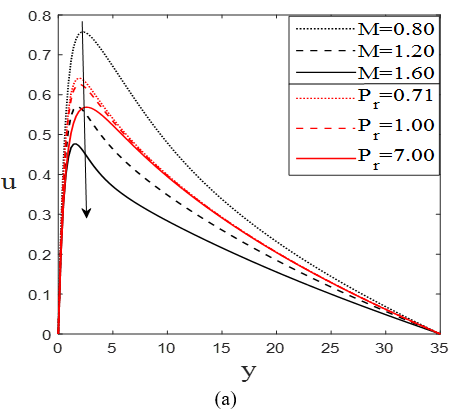## 5b.png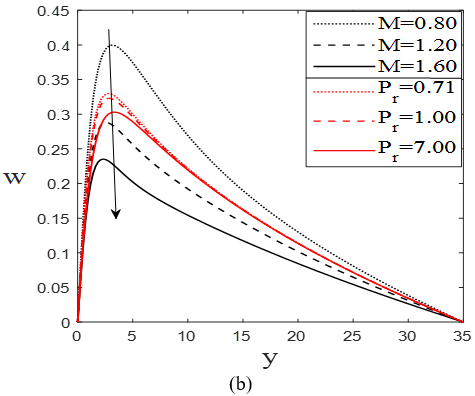## 5c.png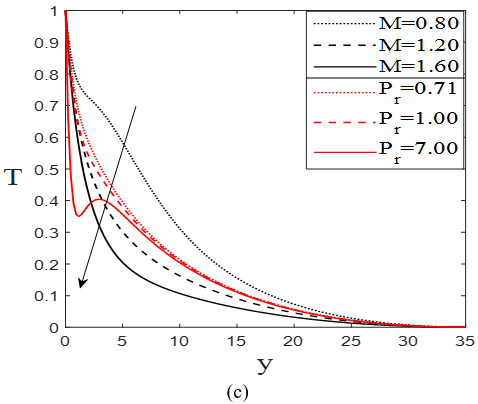Figure 5. Impacts of different values of (M) and (Pr) on (a) primary velocity, (b) secondary velocity, and (c) temperature profile at the time t=120 (steady-state)

## 6a.png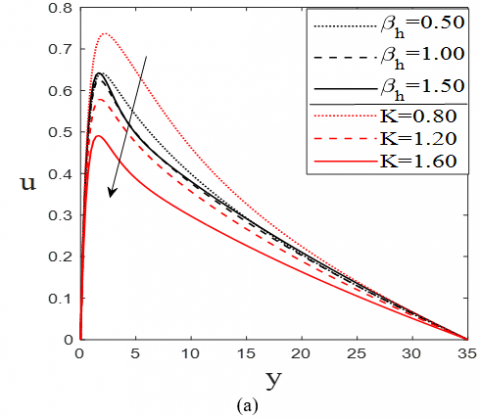## 6b.png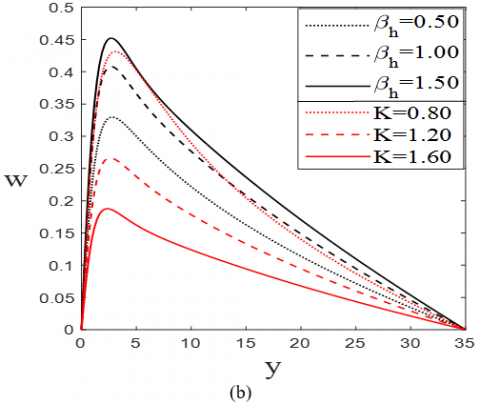## 6c.png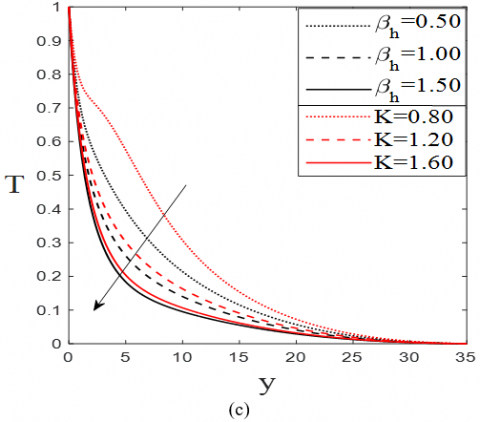Figure 6. Impacts of different values of (βh) and (K) on (a) primary velocity, (b) secondary velocity, and (c) temperature profile at the time t=120 (steady-state)

## 7a.png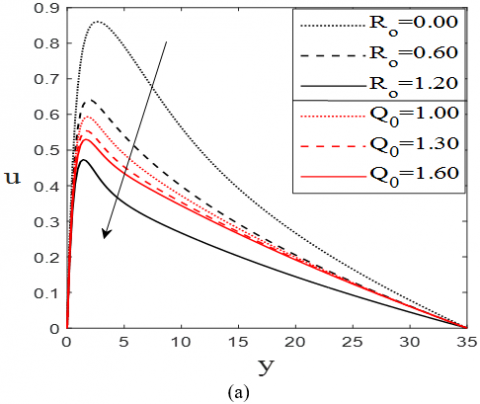## 7b.png## 7c.png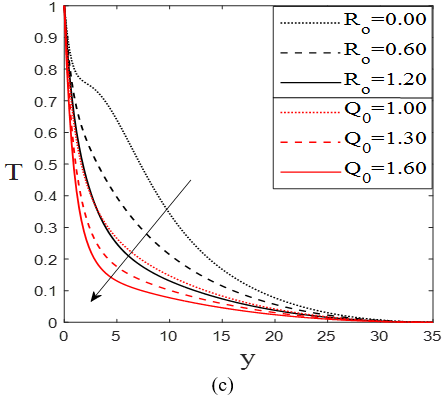Figure 7. Impacts of different values of (Ro) and (Q0) on (a) primary velocity, (b) secondary velocity, and (c) temperature profile at the time t=120 (steady-state)

## 8a.png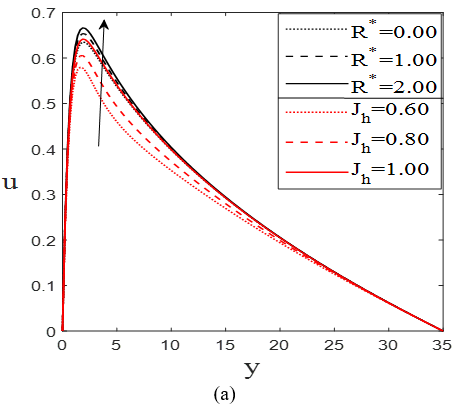## 8b.png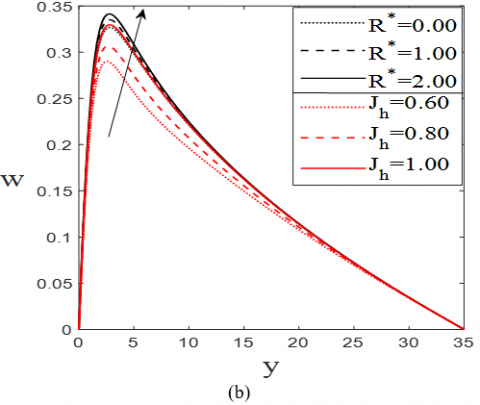## 8c.png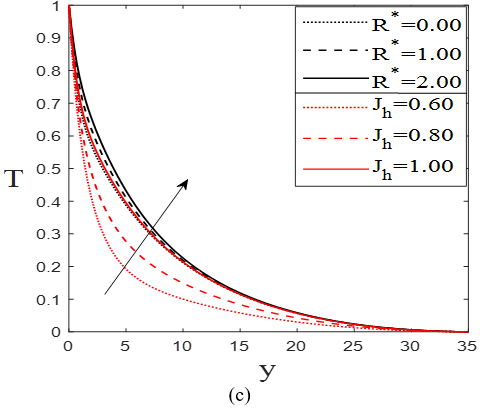Figure 8. Impacts of different values of (R*) and (Jh) on (a) primary velocity, (b) secondary velocity, and (c) temperature profile at the time t=120 (steady-state)

## 9a.png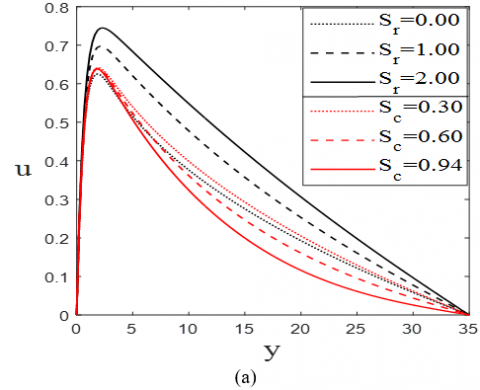## 9b.png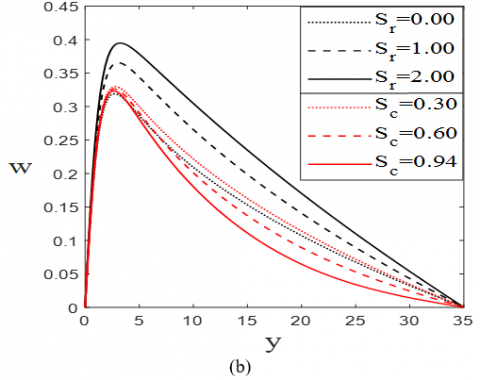## 9c.png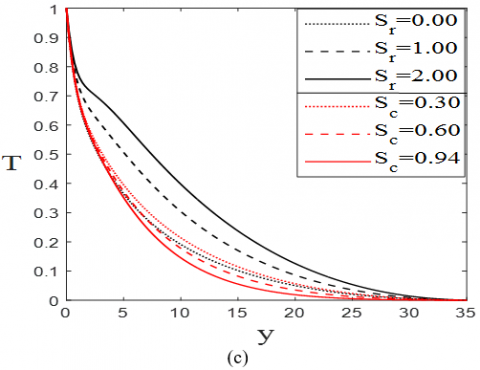## 9d.png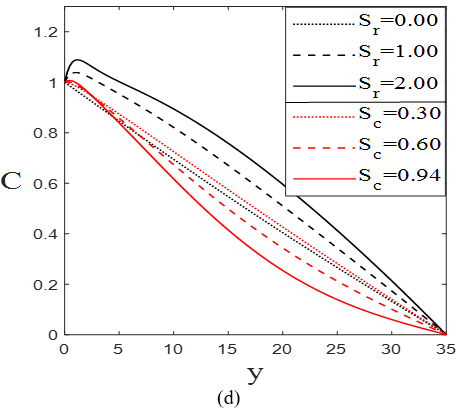Figure 9. Impacts of different values of (Sr) and (Sc) on (a) primary velocity, (b) secondary velocity, (c) temperature profile, and (d) concentration profile at the time t=120 (steady-state)

Table 2. Effects of different parameters on τxL, τzL, NuL and ShL where Eckert number (Ec=0.01)

 Effects of Parameters Profiles Parameters identity Values τxL τzL NuL ShL M 1.00 1.25 1.50 1.0036 0.9303 0.8737 (Dec.) 0.2859 0.2615 0.2441 (Dec.) 0.4276 0.4796 0.5121 (Inc.) 0.0069 0.0038 0.0019 (Dec.) Pr 0.71 1.00 7.00 1.0036 0.9842 0.8431 (Dec.) 0.2859 0.2793 0.2414 (Dec.) 0.4276 0.5226 1.4649 (Inc.) 0.0069 0.0012 -0.0554 (Dec.) K 1.00 1.25 1.50 1.0036 0.9359 0.8816 (Dec.) 0.2859 0.2363 0.2007 (Dec.) 0.4276 0.4802 0.5135 (Inc.) 0.0069 0.0038 0.0018 (Dec.) βh 0.50 1.00 1.50 1.0036 1.0143 1.0429 (Inc.) 0.2859 0.3546 0.3858 (Inc.) 0.4276 0.5014 0.5485 (Inc.) 0.0069 0.0025 -0.0003 (Dec.) Ro 0.00 0.60 1.20 1.1328 1.0036 0.8675 (Dec.) 0.1394 0.2859 0.3625 (Inc.) 0.3356 0.4276 0.4948 (Inc.) 0.0123 0.0069 0.0029 (Dec.) Qo 0.80 1.20 1.60 1.0036 0.9425 0.9082 (Dec.) 0.2859 0.2598 0.2467 (Dec.) 0.4276 0.6459 0.7886 (Inc.) 0.0069 -0.0061 -0.0147 (Dec.) Jh 0.60 0.80 1.00 0.9630 0.9807 1.0036 (Inc.) 0.2662 0.2747 0.2859 (Inc.) 0.5381 0.4893 0.4276 (Dec.) 0.0003 0.0032 0.0069 (Inc.) R* 0.00 1.00 2.00 0.9959 1.0169 1.0298 (Inc.) 0.2833 0.2906 0.2954 (Inc.) 0.4642 0.3658 0.3092 (Dec.) 0.0047 0.0106 0.0140 (Inc.) Sr 0.00 1.00 2.00 0.9923 1.0417 1.0767 (Inc.) 0.2807 0.3031 0.3181 (Inc.) 0.4384 0.3900 0.3562 (Dec.) 0.0308 -0.0780 -0.1669 (Dec.) Sc 0.30 0.60 0.94 1.0036 1.0051 1.0071 (Inc.) 0.2859 0.2857 0.2858 0.4276 0.4301 0.4321 (Inc.) 0.0069 -0.0076 -0.0239 (Dec.)
7. Conclusions

A worthwhile endeavor to observe thermal radiation, Hall current along with heat generation/absorption, and Joule heating effects on unsteady flow pattern have been represented in this paper. Also, the imposition of the negative system rotation with a constant angular velocity through a non-oscillating medium of porosity under the dominance of a uniform magnetic field is mentioned. The wielding of the fluid happens due to the body force created by the magnetic field. All the relevant parameters’ effect on the flow behavior have been discussed as well as appropriate mesh space and steady-state of the solutions are also constructed. The most significant upshots of this mathematical investigation are arranged below:

(1) The steady-state solutions are confirmed at the time t=120.

(2) The appropriate cell size for the execution of numerical calculations is taken as (m, n)=(150, 150).

(3) Primary velocity increasingly varies with the increment of Jh, Sr, and R* while it retards by the rise of M, βh, Pr, K, Ro, Sc, and Q0.

(4) M, Pr, K, Sc, and Q0 reduce secondary velocity while Jh, βh, Sr, and R0 augment secondary velocity.

(5) With the enhancement of Jh, R*, and Sr a raising effect is marked on temperature distribution, on contrary with the prolongation values of M, βh, Pr, K, R0, Sc, and Q0 a reducing effect is reckoned on the temperature profile.

(6) With the amplifying values of Sr concentration profile enlarges while Sc makes it shorten.

(7) Enhancement on local primary shear stress is checked with increasing values of Jh, βh, Sr, Sc, and R* while M, Pr, K, R0, and Q0 resist it.

(8) The local secondary shear stress promotes with Jh, βh, Sr, R0, and R* whereas M, Pr, K, and Q0 causes retardation on local secondary shear stress.

(9) Local Nusselt number raises with M, Pr, K, R0, βh, Sc, and Q0 on contrary, it collapses with Jh, Sr and R*.

(10) Local Sherwood number raises with Jh and R*, and it declines with M, Pr, K, R0, βh, Sc, Sr, and Q0.

Nomenclature
 u*, w* Cartesian primary and secondary velocity components x*, y* Cartesian components in the plate’s and normal to the plate’s direction $T_{w}^{*}, C_{w}^{*}$ temperature and concentration at the plate $T_{\infty}^{*}, C_{\infty}^{*}$ temperature and concentration at the quiescent region B0 uniform magnetic field Ωr uniform angular velocity cp specific heat at constant pressure k* mean absorption coefficient g acceleration due to gravity L characteristic length u, w dimensionless primary and secondary velocity components x, y dimensionless Cartesian co-ordinates m, n number of cells in x and y directions $T_{m}^{*}$ mean fluid temperature D* mass diffusivity coefficient kp porous medium permeability M magnetic parameter Pr Prandtl number K permeability parameter Jh Joule heating parameter Ro rotational parameter Qo heat changing parameter Ec Eckert number Sr Soret number Sc Schmidt number R* radiation parameter NuL, ShL local Nusselt and local Sherwood number Greek symbols υ kinematic viscosity σ electrical conductivity β Coefficient of thermal expansion β* Coefficient of concentration expansion κ thermal conductivity σ* Stefan-Boltzman constant τxL, τzL local primary and local secondary shear stresses βh Hall parameter Subscripts w Condition at plate ∞ Condition at the quiescent region
References

 Sattar, M.A., Alam, M.M. (1992). Unsteady hydromagnetic free convection flow with Hall current and mass transfer along an accelerated porous plate with time dependent temperature and concentration. Canadian Journal of Physics, 70(5): 369-374. https://doi.org/10.1139/p92-061

 Biswal, S., Sahoo, P.K. (1994). Hall effect on oscillatory hydro-magnetic free convective flow of a visco-elastic fluid past an infinite vertical porous flat plate with mass transfer. Proc. Nat. Acad. Sci, 69A: 46-52.

 Kafoussias, N.G., Williams, E.M. (1995). Thermal-diffusion and diffusion-thermo effects on mixed free-forced convective and mass transfer boundary layer flow with temperature dependent viscosity. International Journal of Engineering Science, 33(9): 1369-1384. https://doi.org/10.1016/0020-7225(94)00132-4

 Takhar, H.S., Jha, B.K. (1998). Effects of hall and ion-slip currents on MHD flow past an impulsively started plate in a rotating system. Journal of Magnetohydrodynamics and Plasma Research, 8: 61-72.

 Barletta, A., Rossi di Schio, E. (2001). Effect of viscous dissipation on mixed convection heat transfer in a vertical tube with uniform wall heat flux. Heat and Mass Transfer Journal, 38(1-2): 129-140. https://doi.org/10.1007/s002310100204

 Anwar Be´g, O., Be´g, T.A., Takhar, H.S., Raptis, A. (2004). Mathematical and numerical modeling of non-Newtonian thermo-hydrodynamic flow in non-Darcy porous media. International Journal of Fluid Mechanics Research, 31(1): 1-12. https://doi.org/10.1615/InterJFluidMechRes.v31.i1.10

 Duwairi, H.M. (2005). Viscous and Joule heating effects on forced convection flow from radiate isothermal porous surfaces. International Journal of Numerical Methods for Heat and Fluid Flow, 15(5): 429-440. https://doi.org/10.1108/09615530510593620

 Alam, M.S., Rahman, M.M., Samad, M.A. (2006). Numerical study of the combined free-forced convection and mass transfer flow past a vertical porous plate in a porous medium with heat generation and thermal diffusion. Nonlinear Analysis: Modeling and Control, 11(4): 331-343. https://doi.org/10.15388/NA.2006.11.4.14737

 Reza-E-Rabbi, S., Ahmmed, S.F., Arifuzzaman, S.M., Sarkar, T., Khan, M.S. (2019). Computational modeling of multiphase fluid flow behaviour over a stretching sheet in the presence of nanoparticles. Engineering Science and Technology, an International Journal, 23(3): 605-617. https://doi.org/10.1016/j.jestch.2019.07.006

 Sharma, B.K., Jha, A.K., Chaudhary, R.C. (2007). Hall effect on MHD mixed convective flow of a viscous incompressible fluid past a vertical porous plate immersed in porous medium with heat source/sink. Romanian Journal of Physics, 52(5–7): 487–503.

 Alam, M.S., Rahman, M.M., Sattar, M.A. (2008). Effects of variable suction and thermophoresis on steady MHD combined free-forced convective heat and mass transfer flow over a semi–infinite permeable inclined plate in the presence of thermal radiation. International Journal of Thermal Science, 47(6): 758-765. https://doi.org/10.1016/j.ijthermalsci.2007.06.006

 Bakier, A.Y., Rashed, A.M., Mansour, M.A. (2009). Group method analysis of melting effect on MHD mixed convection flow from radiate vertical plate embedded in a saturated porous media. Communications in Nonlinear Science and Numerical Simulation, 14(5): 2160-2170. https://doi.org/10.1016/j.cnsns.2008.06.016

 Pal, D., Talukdar, B. (2010). Buoyancy and chemical reaction effects on MHD mixed convection heat and mass transfer in a porous medium with thermal radiation and Ohmic heating. Communications in Nonlinear Science and Numerical Simulation, 15(10): 2878-2893. https://doi.org/10.1016/j.cnsns.2009.10.029

 Alam, M.M., Hossain, M.D., Hossain, M.R. (2011). Viscous dissipation and Joule heating effects on steady MHD combined heat and mass transfer flow through a porous medium in a rotating system. Journal of Naval Architecture and Marine Engineering, 8(2): 105-120. https://doi.org/10.3329/jname.v8i2.5055

 Islam, T., Islam M.M. (2020). MHD mixed convective heat transfer of a micropolar fluid over an unsteady stretching porous wedge with viscous dissipation and Joule heating. Journal of Applied Mathematics and Statistical Analysis, 1(1): 1–12. https://doi.org/10.5281/zenodo.3859468

 Reddy, T.S., Reddy, O.S.P., Raju, M.C., Varma, S.V.K. (2012). Heat transfer in hydromagnetic rotating flow of viscous fluid through non-homogeneous porous medium with constant heat source/sink. International Journal of Mathematical Archive, 3(8): 2964–2973.

 Hasan, M.S., Mondal, R.N., Lorenzini, G. (2020). Physics of bifurcation of the flow and heat transfer through a curved duct with natural and forced convection. Chinese Journal Physics, 67: 428-457. https://doi.org/10.1016/j.cjph.2020.07.004

 Seth, G.S., Nandkeolyar, R., Ansari, M.S. (2013). Effects of thermal radiation and rotation on unsteady hydromagnetic free convection flow past an impulsively moving vertical plate with ramped temperature in a porous medium. Journal of Applied Fluid Mechanics, 6(1): 27-38. https://doi.org/10.36884/jafm.6.01.19478

 Rashad, A.M., Abbasbandy, S., Chamkha, A.J. (2014). Non-Darcy natural convection from a vertical cylinder embedded in a thermally stratified and nanofluid saturated porous media. Journal of Heat Transfer, 136(2): 1-9. https://doi.org/10.1115/1.4025559

 Seth, G.S., Kumbhakar, B., Sarkar, S. (2014). Unsteady hydromagnetic natural convection flow with heat and mass transfer of a thermally radiating and chemically reactive fluid past a vertical plate with Newtonian heating and time dependent free-stream. International Journal of Heat and Technology, 32(1-2): 87-94. https://doi.org/10.18280/ijht.320113

 Raju, M.C., Varma, S.V.K., Reddy, N.A. (2012). Radiation and mass transfer effects on a free convection flow through a porous medium bounded by a vertical surface. Journal of Future Engineering and Technology, 7(2): 7-12. https://doi.org/10.26634/jfet.7.2.1757

 Singh, J.K., Rohidas, P., Joshi, N., Begum, S.G. (2017). Influence of Hall and ion-slip currents on unsteady MHD free convective flow of a rotating fluid past an oscillating vertical plate. International Journal of Heat and Technology, 35(1): 37-52. https://doi.org/10.18280/ijht.350106

 Izadi, M., Shahivand, I., Mehryan, S.A., Hasan, M.S., Lorenzini, G. (2020). Magneto-hydrodynamic flow of micropolar nanofluid containing motile microorganisms passing over a vertical stretching sheet with magnetic field dependent viscosity. Journal of Engineering Thermophysics, 29(4): 632-656. https://doi.org/10.1134/S1810232820040116

 Veera Krishna, M., Swarnalathamma, B.V., Chamkha, Ali J. (2019). Investigations of Soret, Joule and Hall effects on MHD rotating mixed convective flow past an infinite vertical porous plate. Journal of Ocean Engineering and Science, 4(3): 263-275. https://doi.org/10.1016/j.joes.2019.05.002

 Islam, A., Islam, M.M., Rahman, M., Ali, L.E., Khan, M.S. (2016). Unsteady heat transfer of viscous incompressible boundary layer fluid flow through a porous plate with induced magnetic field. Journal of Applied Mathematics and Physics, 4(2): 294-306. https://doi.org/10.4236/jamp.2016.42037

 Islam, M.M., Mollah, M.T., Hasan, M.S., Alam, M.M. (2017). Numerical solution of unsteady viscous compressible fluid flow along a porous plate with induced magnetic field. Modelling, Measurement and Control B, 86(4): 850-863. https://doi.org/10.18280/mmc_b.860403

 Mollah, M.T., Islam, M.M. (2018). Numerical study for compressible fluid with induced magnetic field. Lambert Academic Publishing, Riga, Latvia. ISBN: 978-613-9-93063-0.

 Mollah, M.T., Islam, M.M., Khatun, S., Alam, M.M. (2019). MHD generalized Couette flow and heat transfer on Bingham Fluid through porous parallel plates. Mathematical Modeling of Engineering problems, 6(4): 483-490. https://doi.org/10.18280/mmep.060402

 Mollah, M.T. (2019). EMHD laminar flow of Bingham fluid between two parallel Riga plates. International Journal of Heat and Technology, 37(2): 641-648. https://doi.org/10.18280/ijht.370236

 VeeraKrishna, M., Chamkha, A.J. (2018). Hall effects on unsteady MHD flow of second grade fluid through porous medium with ramped wall temperature and ramped surface concentration. Physics of Fluids, 30(5): 053101. https://doi.org/10.1063/1.5025542

 Anika, N.N., Hoque, M.M., Islam, N. (2013). Hall current effects on Magnetohydrodynamics fluid over an infinite rotating vertical porous plate embedded in unsteady laminar flow. Annals of Pure and Applied Mathematics, 3(2): 189-200.

 Perven, R., Alam, M.M. (2015). MHD free convection fluid flow through parallel plates with hall current in a rotating system. Procedia Engineering, 105: 207-214. https://doi.org/10.1016/j.proeng.2015.05.095

 Mollah, M.T., Poddar S., Islam, M.M., Alam, M.M. (2021). Non-isothermal Bingham fluid flow between two horizontal parallel plates with Ion-slip and Hall currents. SN Applied Sciences, 3: 115 https://doi.org/10.1007/s42452-020-04012-2

 Iva, L.M., Hasan, M.S., Paul, S.K., Mondal, R.N. (2018). MHD free convection heat and mass transfer flow over a vertical porous plate in a rotating system with Hall current, heat source and suction. International Journal of Advances in Applied Mathematics and Mechanics, 6(1): 49-64.